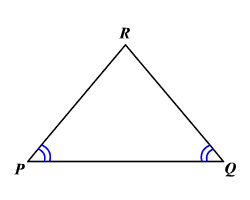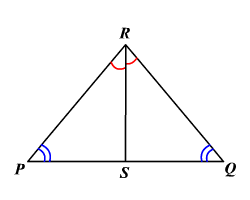# Converse of Isosceles Triangle Theorem

If two angles of a triangle are congruent , then the sides opposite to these angles are congruent.Proof

Draw $\stackrel{¯}{SR}$ , the bisector of the vertex angle $\angle PRQ$ .Since $\stackrel{¯}{SR}$ is the angle bisector , $\angle PRS\cong \angle QRS$ .

By the Reflexive Property ,

$\stackrel{¯}{RS}\cong \stackrel{¯}{RS}$

It is given that $\angle P\cong \angle Q$ .

Therefore, by AAS congruent, $\Delta PRS\cong \Delta QRS$ .

Since corresponding parts of congruent triangles are congruent,

$\stackrel{¯}{PR}\cong \stackrel{¯}{QR}$